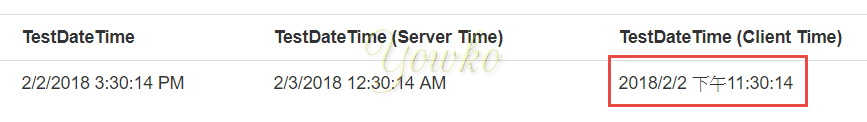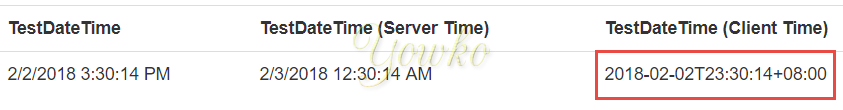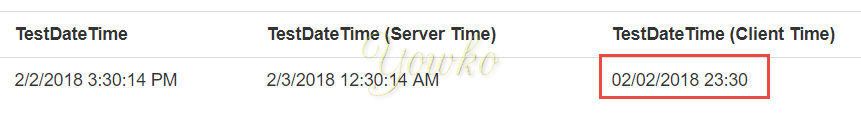# C# DateTime 轉 JavaScript Date (依使用者偏好區域來顯示時間)

## 概念

1. C# 取得 DateTime 資料傳給 JavaScript
2. JavaScript 將原 DateTime 轉為 user 所在時區時間
3. 將轉換完成的時間呈現在網頁上

## 1. C# 取得 DateTime 資料傳給 JavaScript

1. 將目標時間與 js 的啟始時間 (1970/1/1 0:0:0) 兩者時間差轉為毫秒，傳給 js ，再透過 `new Date(總毫秒數)` 取得

• c#

``````var _target=sourceDatetime.Subtract(new DateTime(1970,1,1,0,0,0,DateTimeKind.Utc)).TotalMilliseconds;
``````
• js

``````var dt = new Date(_target);
``````
2. 將 C# DateTime 轉為 js 可直接的格式

• C# (下列兩者皆可)

• ToString(“R”) : `Fri, 02 Feb 2018 15:30:14 GMT`

``````sourceDatetime.ToString("R");
``````
• ToString(“u”):`2018-02-02 15:30:14Z`

``````sourceDatetime.ToString("u");
``````
• js

```js var dt = new Date(targetDatetime); ````

3. 搭配 moment.js

• C# (與 js 相同)

• ToString(“R”) : `Fri, 02 Feb 2018 15:30:14 GMT`

``````sourceDatetime.ToString("R");
``````
• ToString(“u”):`2018-02-02 15:30:14Z`

``````sourceDatetime.ToString("u");
``````
• moment.js

``````var dt = moment(targetDatetime);
``````

## 2. JavaScript 將原 DateTime 轉為 user 所在時區時間

1. js

``````var result=dt.toLocaleString();
``````
2. moment.js

``````var result=dt.local().format();
``````

## 3. 將轉換完成的時間呈現在網頁上

`````` \$('selector').html(result);
``````

## 其他

• moment.js 格式化

``````var result=dt.local().format('DD/MM/YYYY HH:mm')
``````

## 完整程式碼

1. html(cshtml)

由 Server render 指定的 datetime string

• `R`

``````<div id="waitchange" data-val="Fri, 02 Feb 2018 15:30:14 GMT"></div>
``````
• `u`

``````<div id="waitchange" data-val="2018-02-02 15:30:14Z"></div>
``````
• JavaScript

截圖內容說明：

``````*   `TestDateTime` 表示 DB 儲存 UTC 時間
*   `TestDateTime (Server Time)` 表 UTC 時間`.ToLocalTime()`
*   `TestDateTime (Client Time)` 為 JS 處理結果
``````
• 原生 js

``````var _element = \$('#waitchange');
var dt = new Date(\$(_element).data('val'));
var result=dt.toLocaleString();
\$(_element).html(result);
``````• 使用 moment.js

``````var _element = \$('#waitchange');
var dt = moment(\$(_element).data('val'));
var result=dt.local().format();
\$(_element).html(result);
``````• 使用 moment.js 格式化輸出

``````var _element = \$('#waitchange');
var dt = moment(\$(_element).data('val'));
var result=dt.local().format('DD/MM/YYYY HH:mm');
\$(_element).html(result);
``````## 心得

1. C# 與 js 之間的 datetime 轉換，有特定格式的要求
2. js date 顯示的格式微調，有 moment.js 會簡單得多